Let's do another one:

 Graph2 thingsand2 sentences!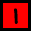The y-intercept:  Find f(0)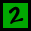The x-intercept:  numerator = 0, solve.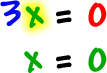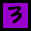Vertical asymptotes:  denominator = 0, solve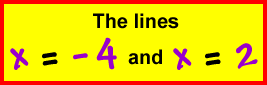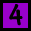Horizontal asymptote:

 Look at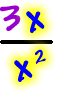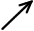* When this is the case, we're going to be forced to "quickie plot" a few points to nail the graph.  No, this isn't being a sissy -- we'll have no choice.  But, Calculus will fix this problem by telling us where the graphs are increasing and decreasing!